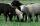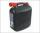Three numbers

What is three numbers which have the property: the sum of the reciprocals of the first and second numbers is 12/7, the first and third 11/24 and the second and the third 3/8.

Result

a =  3
b =  4
c =  8

Solution:A+B = 7/12
A+C = 11/24
B+C = 3/8

12A+12B = 7
24A+24C = 11
8B+8C = 3

A = 13 ≈ 0.333333
B = 14 = 0.25
C = 18 = 0.125

Calculated by our linear equations calculator.Leave us a comment of example and its solution (i.e. if it is still somewhat unclear...):Be the first to comment!To solve this example are needed these knowledge from mathematics:

Do you have a system of equations and looking for calculator system of linear equations?

Next similar examples:

1. Theorem proveWe want to prove the sentence: If the natural number n is divisible by six, then n is divisible by three. From what assumption we started?
2. Three workshopsThere are 2743 people working in three workshops. In the second workshop works 140 people more than in the first and in third works 4.2 times more than the second one. How many people work in each workshop?
3. Three unknownsSolve the system of linear equations with three unknowns: A + B + C = 14 B - A - C = 4 2A - B + C = 0
4. Lord RamWhen lord Ram founded the breed white sheep was 8 more than black. Currently white sheep are four times higher than at the beginning and black three times more than at the beginning. White sheep is now 42 more than the black. How many white and black sh
5. Three friendsThe three friends spent 600 KC in a teahouse. Thomas paid twice as much as Paul. Paul a half less than Zdeněk. How many each paid?
6. Gasoline35 l of gasoline is to be divided into four canisters so that in the third canister was 5 l less than the first canister, the fourth canister 10 l more than in the third canister and the second canister half what in the first canister. How many liters of.
7. Father 7Father is 6 times older than his son. After 4 years, the father will only be 4 times older. What are their present ages?
8. LegsCancer has 5 pairs of legs. The insect has 6 legs. 60 animals have a total of 500 legs. How much more are cancers than insects?
9. CandiesIf Alena give Lenka 3 candy will still have 1 more candy. If Lenka give Alena 1 candy Alena will hame twice more than Lenka. How many candies have each of them?
10. AntennasIf you give me two antennas will be same. If you give me again your two antenna I have a 5× so many than you. How many antennas have both mans?
11. Linsys2Solve two equations with two unknowns: 400x+120y=147.2 350x+200y=144
12. Elimination methodSolve system of linear equations by elimination method: 5/2x + 3/5y= 4/15 1/2x + 2/5y= 2/15
13. BottlesThe must is sold in 5-liter and 2-liter bottles. Mr Kucera bought a total of 216 liters in 60 bottles. How many liters did Mr. Kucera buy in five-liter bottles?
14. A candleA candle shop sells scented candles for \$16 each and unscented candles for \$10 each. The shop sells 28 candles today and makes \$400. a. Write a system of linear equations that represents the situation. b. Solve the system to answer the questions: How m
15. Two equationsSolve equations (use adding and subtracting of linear equations): -4x+11y=5 6x-11y=-5
16. ChildrenThe group has 42 children. There are 4 more boys than girls. How many boys and girls are in the group?
17. Linear systemSolve a set of two equations of two unknowns: 1.5x+1.2y=0.6 0.8x-0.2y=2